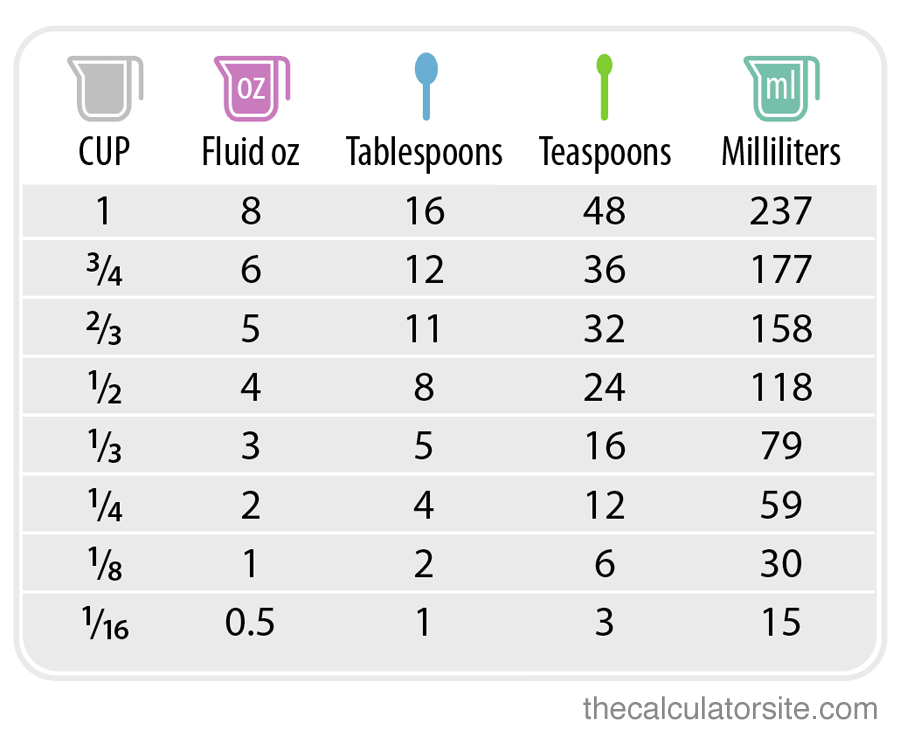# Cups to Oz

OZ to Cups

1 US Fluid Ounce = 0.125 US Cu

## How to convert fluid ounces OZ to Cups?

OZ to Cups 1 Fluid ounce (oz) is equivalent to 0.125 cups. To transform fluid oz to cups, multiply the fluid oz value by 0.125 or divide by 8.

For example, to change 16 fluid ounce  to cups, divide 16 by 8, that makes 2 cups in 16 fluid oz.

US fluid oz to cups formula

cup = fl oz * 0.125

cup = fl oz / 8

Fluid ounce (oz) is equivalent to 0.125 cups. To transform fluid ounce to cups, multiply the fluid oz unit by 0.125 or divide by 8. For example, to convert 16 fluid ounce to cups, divide 16 by 8, that makes 2 cups in 16 fluid oz.

### Teaspoons

 Teaspoons Drops Tablespoons Milliliters* 1/2 teaspoon 30 drops 1/6 tablespoon 2.5 milliliters 1 teaspoon 60 drops 1/3 tablespoon 5 milliliters

#### What is a Fluid Ounce?

The fluid ounce is an imperial and United States Customary computation system volume unit. The symbol is “fl oz”.

Table of (fluid) ounce to US cups:

• 1 oz = 1/8 cup
• 2 oz = 1/4 cup
• 3 oz = 3/8 cup
• 4 oz = 1/2 cup
• 6 oz = 3/4 cup
• 8 oz = 1 cup
• 16 oz = 2 cup
• 24 oz = 3 cup
• 32 oz = 4 cup

#### US recipes – cups to ounces

• 1 US cup (236.59mL) = 8 US fluid ounces
• 1 US cup (236.59mL) = 8.327 UK fluid ounces

#### Old UK recipes – cups to ounces

• 1 UK cup (284.13mL) = 10 UK fluid ounces
• 1 UK cup (284.13mL) = 9.607 US fluid ounces

#### Rest of world recipes

• 1 international metric cup (250mL) = 8.454 US fluid ounces
• 1 international metric cup (250mL) = 8.799 UK fluid ounces### 1/2 cup to oz (fluid)

there are 4 ounces is 1/2 cup.

### 1/3 cup to oz (fluid)

there are 2.67 ounces is 1/3 cup.

### 1/4 cup to oz (fluid)

there are 2 ounces in 1/4 cup

Check Also 5 mg to ml

### Fl. Ounce to Cup Conversion Table

Fl. Ounce Cup Fl. Ounce Cup Fl. Ounce Cup Fl. Ounce Cup
1 0.125 26 3.25 51 6.375 76 9.5
2 0.25 27 3.375 52 6.5 77 9.625
3 0.375 28 3.5 53 6.625 78 9.75
4 0.5 29 3.625 54 6.75 79 9.875
5 0.625 30 3.75 55 6.875 80 10
6 0.75 31 3.875 56 7 81 10.125
7 0.875 32 4 57 7.125 82 10.25
8 1 33 4.125 58 7.25 83 10.375
9 1.125 34 4.25 59 7.375 84 10.5
10 1.25 35 4.375 60 7.5 85 10.625
11 1.375 36 4.5 61 7.625 86 10.75
12 1.5 37 4.625 62 7.75 87 10.875
13 1.625 38 4.75 63 7.875 88 11
14 1.75 39 4.875 64 8 89 11.125
15 1.875 40 5 65 8.125 90 11.25
16 2 41 5.125 66 8.25 91 11.375
17 2.125 42 5.25 67 8.375 92 11.5
18 2.25 43 5.375 68 8.5 93 11.625
19 2.375 44 5.5 69 8.625 94 11.75
20 2.5 45 5.625 70 8.75 95 11.875
21 2.625 46 5.75 71 8.875 96 12
22 2.75 47 5.875 72 9 97 12.125
23 2.875 48 6 73 9.125 98 12.25
24 3 49 6.125 74 9.25 99 12.375
25 3.125 50 6.25 75 9.375 100 12.5

How Many Ounces in a Cup

How many oz a cup? = 0.125oz

How many dry Oz are in a cup?

Does 4 ounces equal 1 cup? yes

Is 2.5 oz a cup? yes

How much is 6 oz of a cup? 0.75

What measuring cup is 3 oz?

How tall is a 3 oz cup?

How many spoons is 3 ounces?

How many cups is 3 oz of flour?

Is 4 oz half a cup?

How many oz flour in a cup?

How many cups is 8 oz flour?

12 oz to cup = 1.5 cup
oz to cups dry
6 oz to cup = 0.75 cup
8 oz to cup = 1 cup
oz to cup conversion
4 oz to cup = 0.5 cup
16 oz to cup = 2 Cup
5 oz to cup =0.625 cup

Categories CC